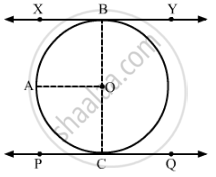# Prove that the Line Segment Joining the Points of Contact of Two Parallel Tangents of a Circle, Passes Through Its Centre. - Mathematics

Prove that the line segment joining the points of contact of two parallel tangents of a circle, passes through its centre.

#### Solution

et XBY and PCQ be two parallel tangents to a circle with centre O.
Construction: Join OB and OC.
Draw OAXYNow, XBAO
XBO + AOB = 180°           (sum of adjacent interior angles is 180°)

Now, XBO = 90°                      (A tangent to a circle is perpendicular to the radius through the point of contact)
⇒ 90° + AOB = 180°
AOB = 180° − 90° = 90°

Similarly, AOC = 90°
AOB + AOC = 90° + 90° = 180°
Hence, BOC is a straight line passing through O.
Thus, the line segment joining the points of contact of two parallel tangents of a circle, passes through its centre.

Concept: Concept of Circle - Centre, Radius, Diameter, Arc, Sector, Chord, Segment, Semicircle, Circumference, Interior and Exterior, Concentric Circles
Is there an error in this question or solution?